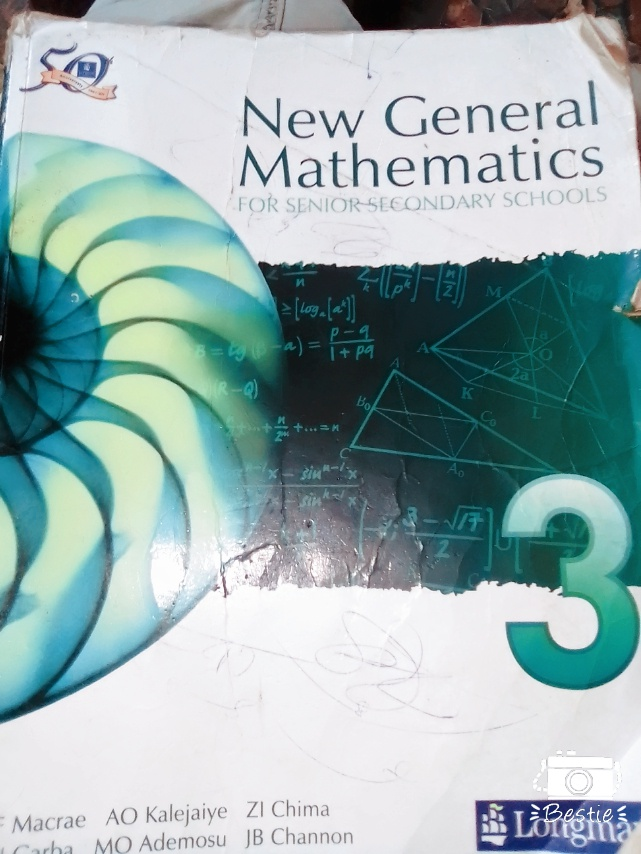Sign inEducation

Examination

#Whenever discussion centres on Mathematics a lot of people become uncomfortable. This is largely as a result of these poor understanding of the basic principles that govean the different topics that make up this important subject.

## In This article I am going to deal with simple linear equations, which are frequently met in SSCE and NECO examinations through worked examples with accompanying easy to understand explanations.

Example 1

Solve the equation

7X +4 = 2X + 19

solution - Step 1

Collect terms involving the unknown (X) on the left hand side and numbers on the right hand side,

7x - 2x =19 -4

=> 5x = 15

step 2

Divide both sides by 5

=> 5x/5 = 15/5

=> x= 3

Equations involving brackets

Example 2

solve the equation

5 (x +1) = 3 (x + 5)

solution

5 ( x +1 ) = 3 (x +5 )

step1

clear brackets by multiplying by out

=> 5x +5 = 3x + 15

step 2

collect the terms in x on the left hand side and numbers on the right hand side

=> 5x - 3 = 15 - 5

=> 2x = 10

step 3

Divide both sides by 2

=> 2x/ 2 = 10/ 2

=> x = 5

Equations Involving fractions

Example 3

solve the equation

x +1/2 = x + 3/ 5

step 1

Clear the fractions by multiplying both sides using 10, the lowest common multiple ( L .C .M ) of 5 and 2

=> 10( X + 1 )/ 2 = 10 (x + 3 )/5

=> 10x + 10 /2 = 10x + 30 / 5

Step 2

Divide the left hand side by 2 and the right hand side by 5

=> 10x + 10 / 2 = 10x +30

=> 5x + 5 = 2x + 6

Step 3

Collect terms in ' x ' on the left hand side and numbers on the right hand side.

=> 5x - 2x = 6 - 5

=> 3x = 1

step 4

Divide both sides by 3

3x / 3 = 1 / 3

=> x = 1/3

Content created and supplied by: Orjiakus (via Opera News )

NECO SSCE